# 32. ELECTROMAGNETIC INDUCTION

## 32.1. Motional emf

Figure 32.1 shows a rod, made of conducting material, being moved with a velocity v in a uniform magnetic field B. The magnetic force acting on a free electron in the rod will be directed upwards and has a magnitude equal to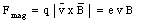(32.1)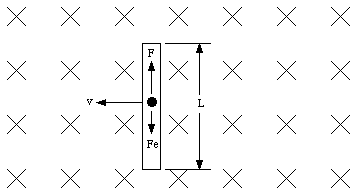Figure 32.1. Moving conductor in magnetic field.

As a result of the magnetic force electrons will start to accumulate at the top of the rod. The charge distribution of the rod will therefore change, and the top of the rod will have an excess of electrons (negative charge) while the bottom of the rod will have a deficit of electrons (positive charge). This charge distribution will produce an electric field in the rod. The strength of this electric field will increase until the electrostatic force produced by this field is equal in magnitude to the magnetic force. As this point the upward flow of electrons will stop and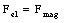(32.2)

or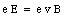(32.3)

The induced electric field will generate a potential difference [Delta]V between the ends of the rod, equal to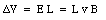(32.4)

where L is the length of the rod. If the ends of the rod are connected with a circuit providing a return path for the accumulated charge, the rod will be a source of emf. Since the emf is associated with the motion of the rod through the magnetic field it is called motional emf. Equation (32.4) shows that the magnitude of the emf is proportional to the velocity v. Looking at Figure 32.1 we observe that vL is the area swept across by the rod per second. The quantity BvL is the magnetic flux swept across by the rod per second. Thus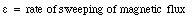(32.5)

Although this formula was derived for the special case shown in Figure 32.1, it is valid in general. It holds for rods and wires of arbitrary shape moving through arbitrary magnetic fields.

Equation (32.5) relates the induced emf to the rate at which the enclosed magnetic flux changes. In the system shown in Figure 32.1 the enclosed flux changes due to the motion of the rod. The enclosed magnetic flux can also be changed if the strength of the enclosed magnetic field changes. In both cases the result will be an induced emf. The relation between the induced emf and the change in magnetic flux is known as Faraday's law of induction:

" The induced emf along a moving or changing mathematical path in a constant or changing magnetic field equals the rate at which magnetic flux sweeps across the path. "

If we consider a closed path, Faraday's law can be stated as follows:

" The induced emf around a closed mathematical path in magnetic field is equal to the rate of change of the magnetic flux intercepted by the area within the path "

or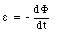(32.6)

The minus sign in eq.(32.6) indicates how polarity of the induced emf is related to the sign of the flux and to the rate of change of flux. The sign of the flux is fixed by the right-hand rule:

" Curl the fingers of your right hand in the direction in which we are reckoning the emf around the path; the magnetic flux is then positive if the magnetic field lines point in the direction of the thumb, and negative otherwise. "

### Example: Problem 32.13

A metal rod of length L and mass m is free to slide, without friction, on two parallel metal tracks. The tracks are connected at one end so that they and the rod form a closed circuit (see Figure 32.2). The rod has a resistance R, and the tracks have a negligible resistance. A uniform magnetic field is perpendicular to the plane of this circuit. The magnetic field is increasing at a constant rate dB/dt. Initially the magnetic field has a strength B0 and the rod is at rest at a distance x0 from the connected end of the rails. Express the acceleration of the rod at this instant in terms of the given quantities.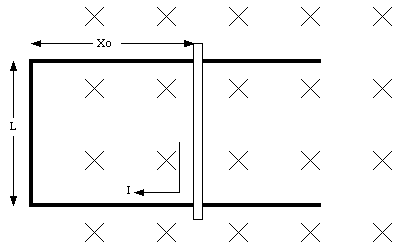Figure 32.2. Problem 32.13.

The magnetic flux [Phi] enclosed by the rod and the tracks at time t = 0 s is given by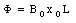(32.7)

The magnetic field is increasing with a constant rate, and consequently the enclosed magnetic flux is also increasing: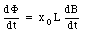(32.8)

Faraday's law of induction can now be used to determine the induced emf: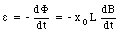(32.9)

As a result of the induced emf a current will flow through the rod with a magnitude equal to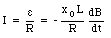(32.10)

The direction of the current is along the wire, and therefore perpendicular to the magnetic field. The force exerted by the magnetic field on the rod is given by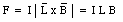(32.11)

(see Chapter 31). Combining eq.(32.10) and (32.11) we obtain for the force on the wire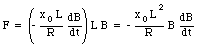(32.12)

The acceleration of the rod at time t = 0 s is therefore equal to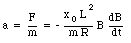(32.13)

### Example: Problem 32.12

a) A long solenoid has 300 turns of wire per meter and has a radius of 3.0 cm. If the current in the wire is increasing at a rate of 50 A/s, at what rate does the strength of the magnetic field in the solenoid increase ?

b) The solenoid is surrounded by a coil with 120 turns. The radius of this coil is 6.0 cm. What induced emf will be generated in this coil while the current in the solenoid is increasing ?

a) The magnetic field in a solenoid was discussed in Chapter 31. If the solenoid has n turns per meter and if I is the current through each coil than the field inside the solenoid is equal to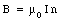(32.14)

Therefore,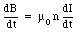(32.15)

In this problem n = 300 turns/meter and dI/dt = 50 A/s. The change in the magnetic field is thus equal to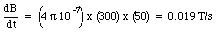(32.16)

This equation shows that the magnetic field is increasing at a rate of 0.019 T/s.

b) Since the magnetic field in the solenoid is changing, the magnetic flux enclosed by the surrounding coil will also change. The flux enclosed by a single winding of this coil is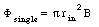(32.17)

where rin = 3.0 cm is the radius of the solenoid. Here we have assumed that the strength of the magnetic field outside the solenoid is zero. The total flux enclosed by the outside coils is equal to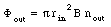(32.18)

The rate of change of the magnetic flux due to that change in magnetic field is given by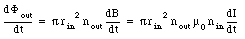(32.19)

As a result of the change in the current in the solenoid an emf will be induced in the outer coil, with a value equal to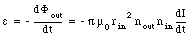(32.20)

If the ends of the coil are connected, a current will flow through the conductor. The direction of the current in the coil can be determined using Lenz' law which states that

" The induced emfs are always of such a polarity as to oppose the change that generates them "

Let us apply Lenz' law to problem 32.12. The direction of the magnetic field can be determined using the right hand rule and is pointed to the right. If the current in the solenoid increases the flux will also increase. The current in the external coil will flow in such a direction as to oppose this change. This implies that the current in this coil will flow counter clock wise (the field generated by the induced current is directed opposite to the field generated by the large solenoid).

## 32.2. The Induced Electric-Field

A rod moving in a magnetic field will have an induced emf as a result of the magnetic force acting on the free electrons. The induced emf will be proportional to the linear velocity v of the rod. If we look at the rod from a reference frame in which the rod is at rest, the magnetic force will be zero. However, there must still be an induced emf. Since this emf can not be generated by the magnetic field, it must be due to an electric field which exists in the moving reference frame. The magnitude of this electric field must be such that the same induced emf is created as is generated in the reference frame in which the rod is moving. This requires that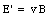(32.21)

The electric field E' that exists in the reference frame of the moving rod is called the induced electric field. The emf generated between the ends of the rod is equal to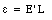(32.22)

which is equivalent to eq.(32.4). If the induced electric field is position dependent, then we have to replace eq.(32.22) with an integral expression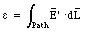(32.23)

where the integral extends from one end of the rod to the other end of the rod.

The difference between the induced electric field and the electric field generated by a static charge distribution is that in the former case the field is not conservative and the path integral along a closed path is equal to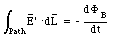(32.24)

which is non-zero if the magnetic flux is time dependent.

## 32.3 Inductance

A changing current in a conductor (like a coil) produces a changing magnetic field. This time-dependent magnetic field can induce a current in a second conductor if it is placed in this field. The emf induced in this second conductor, [epsilon]2, will depend on the magnetic flux through this conductor: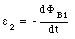(32.25)

The flux [Phi]B1 depends on the strength of the magnetic field generated by conductor 1, and is therefore proportional to the current I1 through this conductor: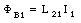(32.26)

Here, the constant L21 depends on the size of the two coils, on their separation distance, and on the number of turns in each coil. The constant L21 is called the mutual inductance of the two coils. Using this constant, eq.(32.25) can be rewritten as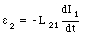(32.27)

The unit of inductance is the Henry (H) and from eq.(32.27) we conclude that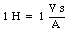(32.28)

When the magnetic field generated by a coil changes (due to a change in current) the magnetic flux enclosed by the coil will also change. This change in flux will induce an emf in the coil, and since the emf is due to a change in the current through the coil it is called the self-induced emf. The self-induced emf is equal to(32.29)

In equation (32.29) L is called the self inductance of the coil. The self-induced emf will act in such a direction to oppose the change in the current.

### Example: Problem 32.32

A long solenoid of radius R has n turns per unit length. A circular coil of wire of radius R' with n' turns surround the solenoid. What is the mutual induction ? Does the shape of the coil of wire matter ?

The field inside the solenoid is assumed to be that of an infinitely long solenoid and has a strength equal to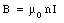(32.30)

The flux enclosed by the external coil is equal to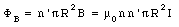(32.31)

The induced emf in the external coil is equal to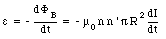(32.32)

The mutual inductance L12 is thus equal to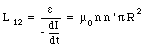(32.33)

## 32.4. Magnetic Energy

If a steady current flows through an inductor, a time-independent magnetic field is created. If suddenly the current source is disconnected, the change in the enclosed magnetic flux will generate a self-induced emf which will try to keep the current flowing in the original direction. The electric energy delivered by the self-induced emf was originally stored in the inductor in the form of magnetic energy. The amount of magnetic energy stored in the magnetic field can be determined by calculating the total power delivered by the power source to create the magnetic field. Suppose that after the battery is connected to the inductor the current increases at a rate of dI/dt. The self-induced emf created by this time-dependent current is equal to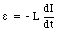(32.34)

The current must deliver extra power to overcome this self-induced emf. The power required will be time dependent and is equal to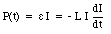(32.35)

The work done by the current is stored in the inductor as magnetic energy. The change dU in the magnetic energy of the inductor is thus equal to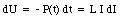(32.36)

The total energy stored in the magnetic field of the inductor when the current reaches its final value can be obtained by integrating eq.(32.36) between I = 0 and I = If.(32.37)

For a solenoid of length l the self-inductance is equal to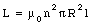(32.38)

The magnetic energy stored in the solenoid is thus equal to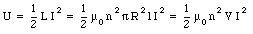(32.39)

where V is the volume of the solenoid. The magnetic energy can be expressed in terms of B and V: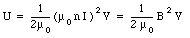(32.40)

where B = u0 n I is the magnetic field in the solenoid. The total magnetic energy of an inductor can now be expressed in terms of the magnetic energy density u which is defined as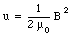(32.41)

The magnetic energy stored in the magnetic field is equal to the energy density time the volume. Although we have derived the formula for the magnetic energy density for the special case of a very long solenoid, its derivation is valid for any arbitrary magnetic field.

### Example: Problem 32.46

A toroid of square cross section has an inner radius R1 and an outer radius R2. The toroid has N turns of wire carrying a current I ; assume that N is very large.

a) Find the magnetic energy density as function of the radius.

b) By integrating the energy density, find the total magnetic energy stored in the solenoid.

c) Deduce the self-inductance from the formula U = L . I 2/2.

a) Apply Ampere's law using a spherical Amperian loop with radius r(32.42)

The current enclosed by the Amperian loop is equal to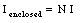(32.43)

Using Ampere's law we can determine the magnetic field B :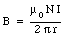(32.44)

The magnetic energy density is thus equal to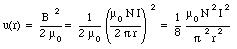(32.45)

b) Suppose the height of the toroid is equal to h. Consider the a slice of the toroid shown in Figure 32.3.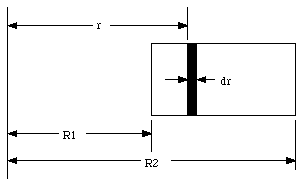Figure 32.3. Cross section of toroid of Problem 32.46.

The volume dV of this slice is equal to(32.46)

The magnetic energy stored in this segment is equal to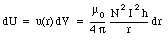(32.47)

The total magnetic energy stored in the toroid can be obtained by integrating eq.(32.47) with respect to r between r = R1 and r = R2: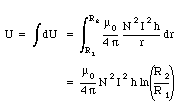(32.48)

c) The magnetic energy stored in an inductor of inductance L is equal to 0.5 L I 2. Comparing this with eq.(32.48) we conclude that the self inductance L of the toroid is equal to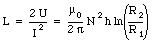(32.49)

## 32.5. The RL circuit

An RL circuit consists of a resistor and an inductor placed in series with a battery (see Figure 32.4). Applying Krichhoff's second rule to this single-loop circuit we obtain the following differential equation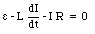(32.50)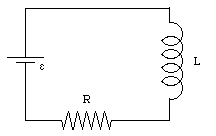Figure 32.4. The RL circuit.

This differential equation has as a solution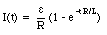(32.51)

This solution is valid if the battery is connected at t = 0. Equation (32.51) shows that the current at t = 0 s is equal to 0 and grows steadily to reach a final value of e /R at t = [infinity]. The time constant of the RL circuit is L/R. If the current has reached a steady value and the battery is suddenly disconnected, the conductor can generate a current through the resistor which will gradually decay as function of time. If the initial current is equal to [epsilon]/R, the current at time t will be equal to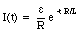(32.52)

### Example: Problem 32.54

What Joule heat is dissipated by the current in eq.(32.52) in the resistor in the time interval between t = 0 and t = [infinity] ? Compare with the initial magnetic energy in the inductor.

The current through the resistor is given in eq.(32.51). The power dissipated by this current in the resistor is equal to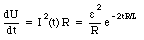(32.53)

The total energy dissipated by this current in the resistor between t = 0 and t = [infinity] is equal to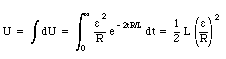(32.54)

The magnetic energy stored in the inductor is equal to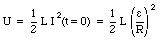(32.55)

and we conclude that all magnetic energy stored in the inductor is dissipated as Joule heat in the resistor.

Send comments, questions and/or suggestions via email to wolfs@nsrl.rochester.edu and/or visit the home page of Frank Wolfs.